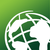# Using geoprocessing results in a widget in Webappbuilder

196
0
04-02-2019 01:09 PMOccasional Contributor

Hi everyone,

I'm trying to get create a geoprocessing service that returns data that I can use in the infographic or chart widget in WAB, but I'm having a tough time doing it. For geoprocessing the user creates a few points on the map, a script then extracts data from rasters at those points. I would like to graph that data, but I can't seem to figure out how to:

A. set up my script properly to create the right data/have the correct environment settings for WAB

B. call the results in the widget

Right now we have the script below working and published, and we have the service set up to return a map service, and we've pointed the chart and infographic back to that service. However whenever we try to chart the results we only get the data that the tool was originally published with, not the most recent results of processing even though the script is working fine and producing unique results.

We are using arcserver 10.5, and the basic version of WAB, this seems like a simple task but we've had a lot of trouble getting it to work right.

Also right now this script creates a crude feature class to hold the resulting data, I'd like to be more efficient and have it in a table, however pointing the widgets back to a resulting table is even murkier than

``````# Start script
#-----------------------------------------------------------------------------
import arcpy
import os

#set environments
arcpy.env.overwriteOutput = True

# Tool initialization
#-----------------------------------------------------------------------------
class Toolbox(object):
def __init__(self):
"""Define the toolbox (the name of the toolbox is the name of the
.pyt file)."""
self.label = "Value_Extraction_Tool"
self.alias = "Value Extraction Tool"

# List of tool classes associated with this toolbox
self.tools = [Tool]

class Tool(object):
def __init__(self):
#Define the tool (tool name is the name of the class)
self.label = "Value Extraction Tool"
self.description = ""
self.canRunInBackground = False

def getParameterInfo(self):
DataSource = arcpy.Parameter(
displayName="DataSource",
name="DataSource",
datatype="DEWorkspace",
parameterType="Required",
direction="Input")

InputPoint = arcpy.Parameter(
displayName="InputPoint",
name="InputPoint",
datatype="DEFeatureClass",
parameterType="Required",
direction="Input")

OutputFC = arcpy.Parameter(
displayName="OutputFC",
name="OutputFC",
datatype="DEFeatureClass",
parameterType="Output",
direction="Output")

params = [DataSource, InputPoint, OutputFC]
return params

# Set whether tool is licensed to execute.
return True

def updateParameters(self, parameters):
# Modify the values and properties of parameters before internal validation is performed.
# This method is called whenever a parameter has been changed.
# parameters.schema.geometryType = "Point"
# parameters.schema.geometryTypeRule = "As Specified"
return

def updateMessages(self, parameters):
#Modify the messages created by internal validation for each tool
#parameter.  This method is called after internal validation.
return

def execute(self, parameters, messages):

# User input arguments
DataSource = parameters.valueAsText
InputPoint = parameters.valueAsText
OutputFC = parameters.valueAsText

# get the projection of the input data
InputSRS = arcpy.Describe(InputPoint).spatialReference

# Projection of the rasters
RasterSRS = arcpy.SpatialReference(4326)

# Create the output feature class
arcpy.CreateFeatureclass_management(os.path.dirname(OutputFC), os.path.basename(OutputFC), "POINT", "", "DISABLED", "DISABLED", InputSRS)

# Add fields to the table to hold the data

# Loop through geometry attributes and get the x and y
with arcpy.da.SearchCursor(InputPoint,['SHAPE@X', 'SHAPE@Y'], spatial_reference = RasterSRS) as cursor:
PointIndex = 1
for row in cursor:
X = row
Y = row

# Get rasters and extract data at an X and Y
index = 0
for (path, dirs, files) in os.walk(DataSource):
for ThisFile in files:
fName,fExt = os.path.splitext(ThisFile)
if fExt.upper() == ".IMG" or fExt.upper() == ".TIF":
RasterPath = path + "\\" + ThisFile
data = (arcpy.GetCellValue_management(RasterPath, str(X) + " " + str(Y), "").getOutput(0))
aquisition_date = arcpy.GetRasterProperties_management(in_raster = RasterPath, property_type = "ACQUISITIONDATE")

if str(aquisition_date).upper() == "UNKNOWN":
insertcursor = arcpy.InsertCursor(OutputFC)
row = insertcursor.newRow()
row.setValue("LABEL", "{}, Point: {}".format(index, PointIndex))
if data.isdigit() == True:
row.setValue("VALUE", int(data))
insertcursor.insertRow(row)
del insertcursor
index += 1

PointIndex += 1
return‍‍‍‍‍‍‍‍‍‍‍‍‍‍‍‍‍‍‍‍‍‍‍‍‍‍‍‍‍‍‍‍‍‍‍‍‍‍‍‍‍‍‍‍‍‍‍‍‍‍‍‍‍‍‍‍‍‍‍‍‍‍‍‍‍‍‍‍‍‍‍‍‍‍‍‍‍‍‍‍‍‍‍‍‍‍‍‍‍‍‍‍‍‍‍‍‍‍‍‍‍‍‍‍‍‍‍‍‍‍‍‍‍‍‍‍‍‍``````
Tags (2)
0 Replies